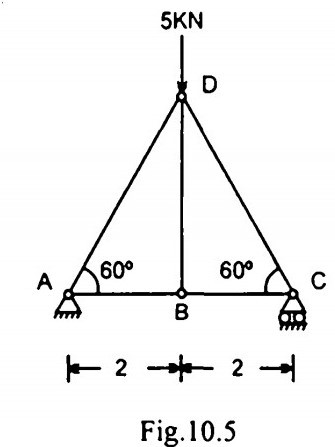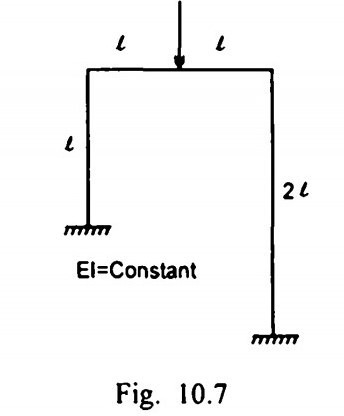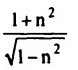Search

# Strength of Materials(101-120)

Updated: Apr 28, 2020

101. A linear arch has normal thrust only

102. A three hinged arch is carrying uniformly distributed load over the entire span. The arch is free from shear force and bending moment if its shape is

parabolic

103. For a determinate pin-jointed plane frame, the relation between the number of joints j and members m is given by m = 2j - 3

104. In the pin-jointed plane frame shown in Fig. 10.5, the force in the member BD iszero

105. The basic perfect frame is a triangle

106. Method of joints is applicable only when the number of unknown forces at the joint under consideration is not more than two

107. Force in the member AD of the pin jointed frame shown in Fig. 10.6 iszero

108. The rigid-jointed plane frame shown in Fig willsway to right

109.A hollow shaft will transmit_________ power than a solid shaft of same weight and material more

110.The stiffness of a helical spring is expressed as load per unit deflection

111.The ratio of maximum shear stress deve loped in a solid shaft of diameter D and a hollow shaft of external diameter D and internal diameter d for the same torque is given by112.If a shaft of diameter d is subjected to a torque, T, the maximum shear stress is

16T/πd³

113.In a rectangular shaft subjected to torsion, the maximum shear stress occurs at

middle of longer side

114.A solid circular shaft of 6m length is built in at its ends and subjected to an

externally applied torque 60 kN-m at a distance of 2 mfromleft end. The reactive

torques at the left end and the right end are respectively

40 kN.m and 20 kN.m

115.The ratio of strain energy stored by a hollow shaft of external diameter D and

internal diameter d and strain energy stored by a solid shaft of diameter D is

(D²+d²)/D²

116.If the internal radius of a hollow shaft is n times the external radius, then ratio of torques carried by the hollow shaft and solid shaft of same cross-sectional area

and subjected to the same maximum shearing stress is117. If a circular shaft is subjected to a torque T and bending moment M, the ratio of maximum bending stress and maximum shear stress is 2M/T

118.If a shaft of diameter d is subjected to a torque T and a bending moment M, the maximum shear stress is119. The radius of gyration of a circle of radius R is equal to R/2

120. A masonry pier ABCD as shown in Fig. 10.8 supports a vertical load W at a point P. The nature of bending stresses at A due to eccentricity of load about x-x axis and y-y respectively arecompressive and tensile